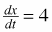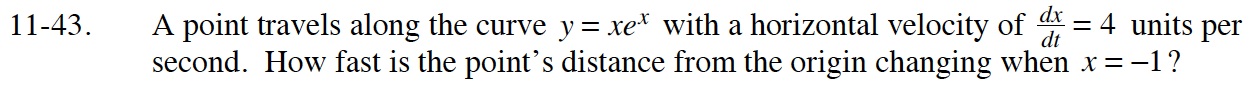### Home > CALC > Chapter 11 > Lesson 11.2.1 > Problem11-43

11-43.

A point travels along the curve y = xex with a horizontal velocity ofunits per second. How fast is the point's distance from the origin changing when x = –1? Homework Help ✎Let d = distance from the origin. Then:

Differentiate:

$2dd^\prime=(2x+2(xe^x)(1e^x+xe^x))x^\prime$

Evaluate when x = –1:

$d=\sqrt{(-1)^2+(-1e^{-1})^2}=\sqrt{1+\frac{1}{e^2}}$

$2\sqrt{1+\frac{1}{e^2}}d^\prime=(2(-1)+2(-1e^{-1})(1e^{-1}-1e^{-1}))(4)$

Solve for d′.